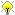[Documentation] [TitleIndex] [WordIndex]Please ask about problems and questions regarding this tutorial on answers.ros.org. Don't forget to include in your question the link to this page, the versions of your OS & ROS, and also add appropriate tags.

# Using Time and TF

Description: This tutorial shows how to use ros::Time and TF to create a tf publisher on the Arduino.

Tutorial Level: INTERMEDIATE

Next Tutorial: Measuring Temperature

Show EOL distros:

## Using Time and TF on the Arduino

The rosserial_arduino package contains libraries for generating timestamps on the Arduino which are synchronized with the PC/Tablet on which the roscore instance is running. This tutorial shows how to access time, using an example of publishing a tf transform.

### The Code

If you have followed the Arduino IDE Setup tutorial, you'll be able to open the sketch below by choosing ros_lib -> TimeTf from the Arduino examples menu.

This should open the following code in your IDE:

```   1 /*
2  * rosserial Time and TF Example
3  * Publishes a transform at current time
4  */
5
6 #include <ros.h>
7 #include <ros/time.h>
9
10 ros::NodeHandle  nh;
11
12 geometry_msgs::TransformStamped t;
14
16 char odom[] = "/odom";
17
18 void setup()
19 {
20   nh.initNode();
22 }
23
24 void loop()
25 {
28   t.transform.translation.x = 1.0;
29   t.transform.rotation.x = 0.0;
30   t.transform.rotation.y = 0.0;
31   t.transform.rotation.z = 0.0;
32   t.transform.rotation.w = 1.0;
35   nh.spinOnce();
36   delay(10);
37 }
```

### The Code Explained

Now, let's break the code down.

```   6 #include <ros.h>
7 #include <ros/time.h>
9
```

We need to include our typical ROS stuff, as well as the transform broadcaster.

```  12 geometry_msgs::TransformStamped t;
14
16 char odom[] = "/odom";
```

Next, we instantiate a TransformStamped message to use, and a broadcaster. We also need to specify the names of the frames we are publishing a transform for.

```  21   broadcaster.init(nh);
```

Inside the setup() function, we have to call init() on the TransformBroadcaster with the node handle as a parameter. Without doing this, the broadcaster will not publish correctly.

```  26   t.header.frame_id = odom;
28   t.transform.translation.x = 1.0;
29   t.transform.rotation.x = 0.0;
30   t.transform.rotation.y = 0.0;
31   t.transform.rotation.z = 0.0;
32   t.transform.rotation.w = 1.0;
```

Inside the loop() function, we fill in the fields of our transform. The frame IDs are set to the correct string names, and the values of the translation and rotation are set.

```  33   t.header.stamp = nh.now();
```

Calling nh.now() returns the current time, as a ros::Time instance, just like when using roscpp's ros::Time::now().

```  34   broadcaster.sendTransform(t);
35   nh.spinOnce();
36   delay(10);
37 }
```

Finally, we publish the transform, and then spinOnce and wait a bit before doing it again. Transforms should always be published at a regular rate, although usually the data fields will be filled in from real data.

### Running the Code

Now, launch the roscore in a new terminal window:

`roscore`

Next, run the rosserial client application that forwards your Arduino messages to the rest of ROS. Make sure to use the correct serial port:

`rosrun rosserial_python serial_node.py /dev/ttyUSB0`

`rosrun rosserial_python serial_node.py _port:=/dev/ttyUSB0`

`rosrun rosserial_python serial_node.py /dev/ttyUSB0`

Finally, you can check the transform using:

`rosrun tf tf_echo odom base_link`

This by default will echo the transform being sent from the Arduino approximately once per second. Note that the Arduino is likely broadcasting at a higher rate (Mega2560 publishes at about 57hz when connected at 57600)

Or, by running:

`rosrun tf view_frames`

This last command will run for about 5 seconds and generate a 'frames.pdf' file in the directory you ran the command in.